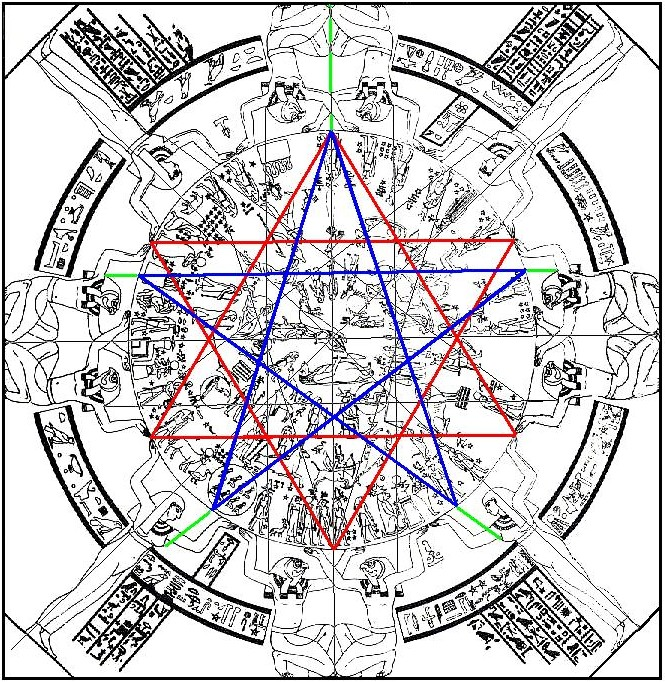The Hidden Geometry of the Zodiac in the Temple of Hathor, Denderah1.        The eight Horus figures holding the circle of the heavens define a pair of crossed axes by the lines defined by knees and elbows.

2.        A hexagram may be drawn in by joining the points where the Isis figures hands meet on the circumference of the circle.

3.        A pair of horizontal lines are drawn as shown by connecting the "shoulders" of the Horus figures, then point where their headresses terminate. These lines occur at the correct location to each form one side of the regular pentagram which can be inscribed in the circle. Thus two pentagrams are formed; one upright and one inverted.

4.        With the remaining lines of the pentagrams drawn in, confirming proof that these constructions represent the intent of the designers is provided by the placement of the straight lines which connect the two fishes of Pisces. Note how one of these two lines not only forms a small section of the side of the pentagram itself, but occurs at the point where this line is intersected by the horizontal diameter of the circle.

Related Pages:

Etheric technology of ancient Egypt: The Temple of Hathor at Denderah